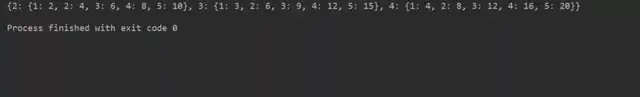字典理解优化

# 字典理解例：
square_dict = {num: num*num for num in range(1, 11)}
print(square_dict)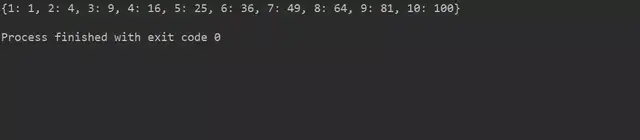三、使用字典理解

dictionary = {key: value for vars in iterable}

1. 图解析语法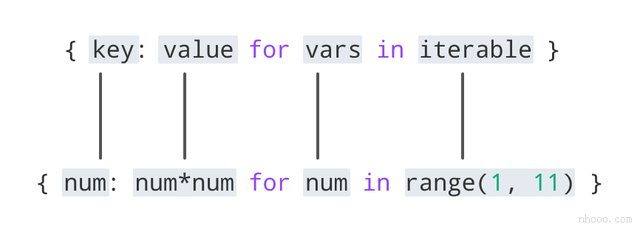如何使用字典理解

#item price in dollars
old_price = {'milk': 1.02, 'coffee': 2.5, 'bread': 2.5}

dollar_to_pound = 0.76
new_price = {item: value*dollar_to_pound for (item, value) in old_price.items()}
print(new_price)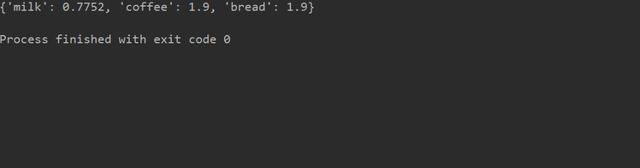1. 如果条件字典理解

original_dict = {'jack': 38, 'michael': 48, 'guido': 57, 'john': 33}

even_dict = {k: v for (k, v) in original_dict.items() if v % 2 == 0}
print(even_dict)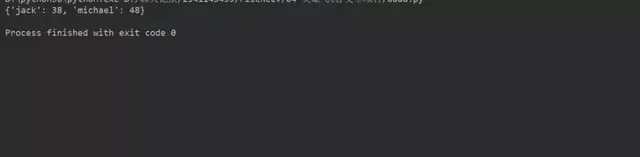2. 多重if条件字典理解

original_dict = {'jack': 38, 'michael': 48, 'guido': 57, 'john': 33}

new_dict = {k: v for (k, v) in original_dict.items() if v % 2 != 0 if v < 40}
print(new_dict)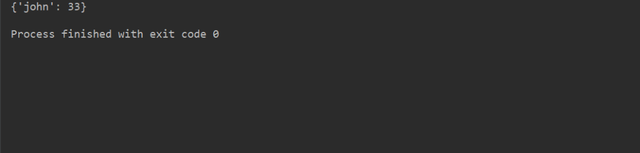3. if-else条件字典理解

original_dict = {'jack': 38, 'michael': 48, 'guido': 57, 'john': 33}

new_dict_1 = {k: ('old' if v > 40 else 'young')
for (k, v) in original_dict.items()}
print(new_dict_1)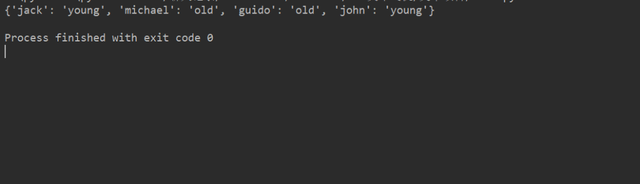具有两个字典理解的嵌套字典

dictionary = {
k1: {k2: k1 * k2 for k2 in range(1, 6)} for k1 in range(2, 5)
}
print(dictionary)